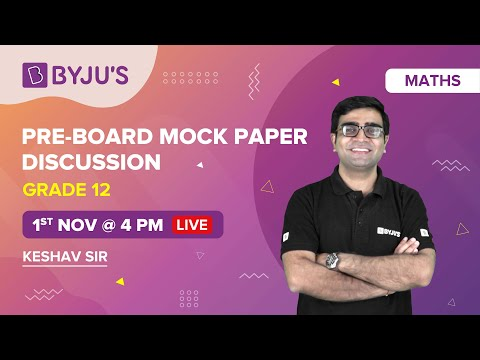# CBSE Class 12 Maths Sample Papers 2023-24 with Solutions PDF

CBSE Sample Papers for Class 12 Maths 2023-24 is the resource without which exam preparation cannot be completed. CBSE Class 12 board exam indicates the end of school life, but it also opens doors to new domains where students can just step in to pursue higher education as per their career desire. Therefore, board exams hold importance in students’ lives as a selection factor for different institutes, colleges and entrance exams that open up a new direction. Students who want to build up their careers in engineering and other streams of Maths must score good marks in Maths subject. The best way to do so is by practising the CBSE Class 12 Sample Papers for Maths. Here, we have provided the latest CBSE Class 12 Maths Sample Paper 2023-24 along with the Marking scheme and Solution PDF of CBSE Sample Paper Class 12 Maths. So, CBSE Class 12 students must practice these Maths Sample Papers to score high marks in the board exam.

## CBSE Sample Paper Class 12 Maths 2023-24 with Solutions

For a better knowledge of the marking scheme and question paper pattern, students can make use of the Sample Paper of 2023-24 for free in PDF form.

Students can download the CBSE Sample Paper for Class 12 Maths 2021-22 Term 2 and 2022-23 exams based on the latest exam pattern from the link below:

#### Watch the below video for CBSE Class 12 Maths Pre-Board Mock PaperTo acquire an extra edge over competitors, try to get a good grip on the basic concepts and fundamentals of the Mathematics subject. Math exam includes different questions based on theorem and formulas, and some of them are asked indirectly in the exam to increase the difficulty level of the paper. So, make sure to revise as many times as possible. The key strategy to ace the CBSE Class 12 Maths exam is continuous practice. Solve different questions of CBSE Sample Paper for Class 12 to get a good grip on Maths subject. Students can also access the sample papers for other subjects of Class 12 by visiting here.

## CBSE Sample Paper Class 12 Maths (Based on Previous Year Pattern)

Students can access the CBSE Class 12 Maths sample paper from the link shown below in the table. After solving the CBSE Sample Paper Class 12 Maths, they can check their answer sheet from the solution file. We have also provided the unsolved CBSE Class 12 Maths Sample Papers. Class 12 students must download them and practice 1 set of CBSE Maths Sample Paper Class 12 daily. These sets of CBSE Sample Paper Class 12 Maths help students to realise their strengths and weakness. After knowing them, they can prepare accordingly for the exam.

#### Access Latest CBSE Sample Paper for Class 12 Maths

CBSE Maths Sample Paper Class 12 Set 1 Access Solution
CBSE Maths Sample Paper Class 12 Set 2
CBSE Maths Sample Paper Class 12 Set 3
CBSE Maths Sample Paper Class 12 Set 4
CBSE Maths Sample Paper Class 12 Set 5
CBSE Maths Sample Paper Class 12 Set 6
CBSE Maths Sample Paper Class 12 Set 7
CBSE Maths Sample Paper Class 12 Set 8
CBSE Maths Sample Paper Class 12 Set 9
CBSE Maths Sample Paper Class 12 Set 10

### CBSE Class 12 Maths Sample Papers Released by CBSE

Every year CBSE releases the CBSE sample papers on its official site to help students understand the question paper pattern and marking scheme of the paper. Here we have compiled the year-wise Maths sample papers. Students can download them from the table below.

CBSE Maths Paper is scoring and less time-consuming as compared to other subjects. Scoring marks in Maths is easy if concepts are strong. Students must write down all the important formulas in one place and paste them into their study room. They should daily go through these formulas so that they will be at their fingertips. Solving different types of questions from the CBSE Maths Sample Paper Class 12 with speed and accuracy will help them in scoring high marks. Also, they get an idea of the types of questions which can be asked in the Maths paper.

We hope students have found this information on “CBSE Sample Paper Class 12 Maths” useful. Keep learning and stay tuned for further updates on CBSE and other competitive exams. Download the BYJU’S App and subscribe to the YouTube channel to access interactive study videos.

## Frequently Asked Questions on CBSE Class 12 Maths Sample Papers

Q1

### What is the pass mark for Class 12 Maths CBSE?

As per the CBSE board, the minimum pass percentage for the Class 12 Maths subject is 33%.

Q2

### Are CBSE Sample QPs and Sample QPs for the Maths subject the same?

Yes, the CBSE Board issues Sample QPs for all subjects every year, and they are named CBSE Sample QPs.

Q3

### How many days of preparation are necessary to score high marks in the Class 12 CBSE Maths board examination?

Students are advised to prepare continuously from Day one for all subjects pertaining to the Board exams. Devoting one hour per subject daily would reduce the burden and stress of last-minute preparation.